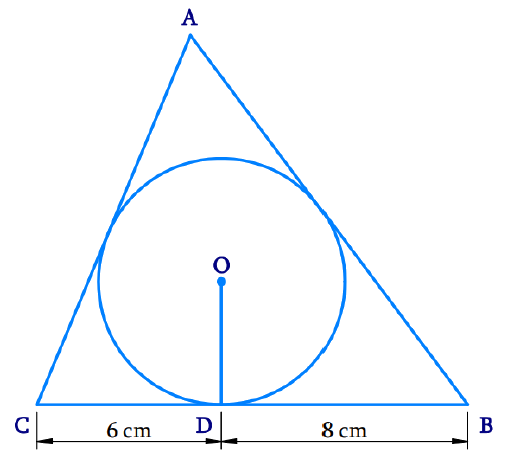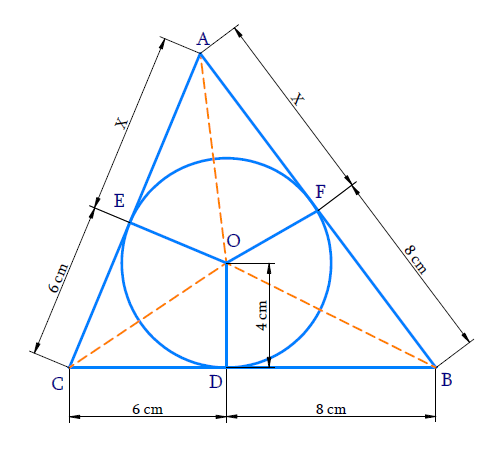# Ex.10.2 Q12 Circles Solution - NCERT Maths Class 10

Go back to  'Ex.10.2'

## Question

A triangle $$ABC$$ is drawn to circumscribe a circle of radius $$4\, \rm{cm}$$ such that the segments $$BD$$ and $$DC$$ into which $$BC$$ is divided by the point of contact $$D$$ are of lengths $$8 \,\rm{cm}$$ and $$6\, \rm{cm}$$ respectively (see Figure). Find the sides $$AB$$ and $$AC$$.Video Solution
Circles
Ex 10.2 | Question 12

## Text Solution

What is the known?

(i) $$\Delta {ABC}$$ is drawn to circumscribe a circle of radius $$4\, \rm{cm.}$$\begin{align}{BD}&=8\;\rm{cm}\\{CD}&=6\;\rm{cm}\end{align}

What is Unknown?

Sides $${AB}$$ and $${AC}$$

Reasoning:

Finding the area of $$\Delta {ABC}$$ in two ways and equating them will result in unknown length. Hence other sides can be calculated.

Let,

$${AE = AF = x}$$ (The lengths of tangents drawn from an external point to a circle are equal.)

$${CD = CE = 6 \rm cm}$$ (Tangents from point $$C$$)

$${BD = BF = 8 \rm{cm}}$$ (Tangents from point $$B$$)

Area of the triangle

\begin{align}= \sqrt {{ s ( s - a ) ( s - b ) ( s - c )} } \end{align}

where \begin{align} s = \frac { 1 } { 2 } ( a + b + c )\end{align}

$$a,\; b$$ and $$c$$ are sides of a triangle

\begin{align} a &= AB= x + 8 \\ b &= BC = 8 + 6 \\ & = 14 \\c& = CA = 6 + x \end{align}

\begin{align} s & = \frac { 1 } { 2 } ( x + 8 + 14 + 6 + x ) \\ s & = \frac { 1 } { 2 } ( 2 x + 28 ) \\ s & = x + 14 \end{align}

Area of $$\Delta {ABC}$$

\begin{align} & = \sqrt { (x + 14) ( x + 14 - x - 8 ) ( x + 14 - 14 ) ( x + 14 - x + 6 ) } \\ & = \sqrt { ( x + 14 ) ( 6 ) ( x ) ( 8 ) } \\ & = \sqrt { 48 x ( x + 14 ) } \\ & = \sqrt { 48 \left( x ^ { 2 } + 14 x \right) }\;\text {sq units}\dots\text{Equation (1)} \end{align}

Area of $$\Delta ABC=$$ Area of $$\Delta {AOC}\;+\;$$Area of $$\Delta {AOB}\;+\;$$ Area of $$\Delta {BOC}$$

\begin{align} & = \frac { 1 } { 2 } ( x + 6 ) 4 + \frac { 1 } { 2 } ( x + 8 ) 4 + \frac { 1 } { 2 } ( 14 ) 4 \\& \left(\therefore \operatorname{area~of} \Delta=\frac{1}{2} \times b \times h\right)\\ & = 2 x + 12 + 2 x + 16 + 28 \\ & = 4 x + 56 \\ & = 4 ( x + 14 ) \text{ sq units}\dots\text{ (Equation 2)} \end{align}

Equating (1) and (2) $\sqrt { 48 \left( x ^ { 2 } + 14 x \right) } = 4 ( x + 14 )$

Squaring both sides

\begin{align} 48 ( x ^ 2 + 14 x ) & = 4 ^ { 2 } ( x + 14 ) ^ { 2 } \\ 48 ( x ^ { 2 } + 14 x ) & = 16 ( x ^ { 2 } + 28 x + 196 ) \\ [\text{Using }(a+b)^{2}&={a^{2}+2 a b+b^{2}}] \\ \frac { 48 } { 16 } ( x ^ { 2 } + 14 x ) & = x ^ { 2 } + 28 x + 196\\ 3 x^{2}+42 x &=x^{2}+28 x+196 \\ \begin{bmatrix}3 x ^ { 2 } - x ^ { 2 } +42 \\ x -28 x - 196 \end{bmatrix} & = 0 \\ 2 x ^ { 2 } + 14 x - 196 & = 0\\ \text{(divide this } & \text{equation by 2)} \\ x ^ { 2 } + 7 x - 98 & = 0 \end{align}

Solving by factorization method

\begin{align} x ^ { 2 } + 14 x - 7 x - 98 & = 0 \\ x ( x + 14 ) - 7 ( x + 14 ) & = 0 \\ ( x + 14 ) ( x - 7 ) & = 0 \end{align}

\begin{align} &{ x + 14 = 0 } \qquad\text{and} \qquad x - 7 = 0 \\ &{ x = - 14 } \qquad \qquad \qquad \;\; { x = 7 } \end{align}

Since $$x$$ represents length, it cannot be negative.

$$\therefore x = 7$$

\begin{align}AB = a = x + 8 = 7 + 8 = 15 \,\rm{cm} \\ AC = c = 6 + x = 6 + 7 = 13\,\rm{cm} \end{align}

Hence the sides

$$AB = 15\, \rm{cm}\\AC = 13 \,\rm{cm}$$

Learn from the best math teachers and top your exams

• Live one on one classroom and doubt clearing
• Practice worksheets in and after class for conceptual clarity
• Personalized curriculum to keep up with school